# Kerala Syllabus 7th Standard Maths Solutions Chapter 12 Squares and Right Triangles

## Kerala State Syllabus 7th Standard Maths Solutions Chapter 12 Squares and Right Triangles

### Squares and Right Triangles Text Book Questions and Answers

Another Way Textbook Page No. 158

There is another way to cut and rejoin two squares of the same size to one large square:Is there any relation between the side of the large square and some length related to the smaller squares?
There is a parallel relationship between the side of the large square and some length related to the smaller squares.

Explanation:
The side of large square = diagonal be d.
Sides of the square be a.
=> Squares of diagonal = two squares of sides.
=> d² = a² × a²How to draw?

When we cut out two squares of the same size and rejoin to make one large square, the length of a side of this large square is length of the diagonal of the small square. So, given a square, it is easy to draw a square of double the area.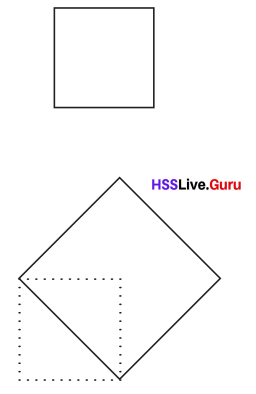Using this idea, can you draw a square of area 50 square centimeters?

We can draw a square of area 50 square centimeters by having the side measurement as 7.07 cms and 5.656 cm for 32 square centimeters.

Explanation:
Area of square = 50 square centimeters.
Area of square = side of the square × side of the square
=> 50 = S²
=> 50 Square root = S
=> 7.07 cm = side of the square.
Area of square = 32 square centimeters.
Area of square = side of the square × side of the square
=> 32 = S²
=> 32 Square root = S
=> 5.656 cm = Side of the square.

Parallel way  Textbook Page No. 160

There is another way to draw a square of double the area of a given square.
First draw a diagonal: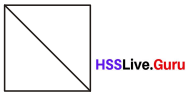Next draw lines parallel to this diagonal through the other two corners:Draw the other diagonal also and draw lines parallel to it as before: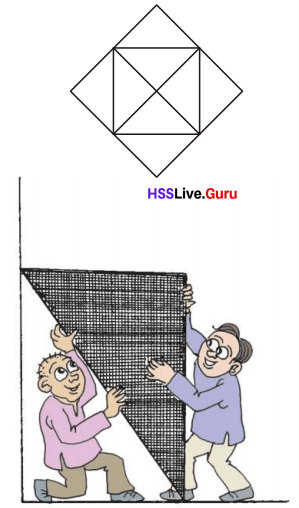How to halve

To get a rectangle of half the area of a square, we need only cut horizontally or vertically along the middle: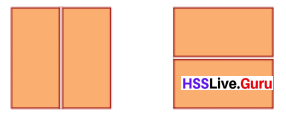Suppose we want a square itself of half the area?
Fold all corners of the square into the Centre:If we now unfold and cut along the creases, We get a square of half the area:Three squares Textbook Page No. 162
We can also cut and join three squares of the same size to make one large square
First cut two squares along the diagonal: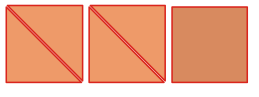Arrange the triangular pieces around the uncut square like this:Draw a square by joining the outer vertices of the triangles as shown below:Cut out the four slender triangles putting out from the square and plug the gaps within: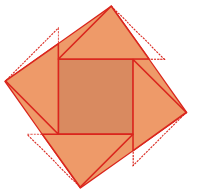Five Squares

Place five squares of the same size like this: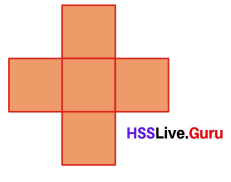Draw a square by joining some of the corners as below: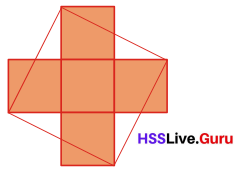Now cut out the triangles outside the square and fill the gaps within: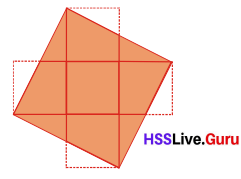Have you seen such a picture in some other lesson?

No, like the above picture and cuttings its not found in any other lessons.

Explanation:
Picture of making five squares from a rectangle is not found in any lessons till now.Another cut Textbook Page No. 164

There are other ways to cut two squares and rearrange to form a single large square.
Mark a side of the small square on one side of the larger square. Join this point with a corner of the square, as shown below:Now place the squares side by side and draw a line as below: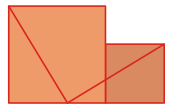Cut along these lines:Move the pieces below to the top as shown below, to make a square:Some history

Abu al-Wafa was a famous mathematician and astronomer who lived in Baghdad during the tenth century AD.One of his books is ‘On Those Parts of Geometry Needed by Craftsmen.” In it, he discusses various methods of joining small squares to make large squares and cutting large squares to make smaller squares.

One such discussion demonstrates that the method used by the craftsmen then to join three squares into one is not accurate and also gives the correct method. It is this method that is given under Three squares in this lesson.

Art and Geometry Textbook Page No. 166

Even before Abu al-Wafa’s time, mosques in Persia were decorated with ornamental tiles on the floors and walls. Abu al-Wafa describes various geometric methods to cut and reassemble such tiles of different sizes.

Many beautiful geometric patterns can be seen in such tiles. The picture below shows such a work on a wall in the famous Janab Abbasi Mosque in Iran, built during the 17th century.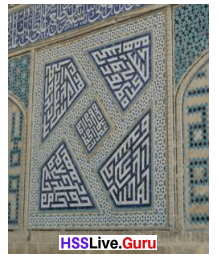Shrinking square

Draw a square of side 5 centimetres and mark points 1 centimetre from the corners as below: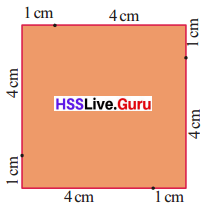We get a square by joining these points:What is its area?
we need only subtract the areas of four right triangles from the area of the large square.
25 – 4 × $$\frac{1}{2}$$ × 4 × 1 = 25 – 8 = 17 sq. cm
Suppose we join points 2 centimetres from the corners.What is the area of the small square?

Area of the small square = 22 square cm.

Explanation:
Area of big square = Side of square × Side of square
= 5 × 5
= 25 square cm.
Area of right triangle = (Side × Height) $$\frac{1}{2}$$
= 2 × 3 × $$\frac{1}{2}$$
= 1 × 3 × $$\frac{1}{1}$$
= 3 square cm.
Area of the small square = Area of big square – Area of right triangle
= 25 – 3
= 22 square cm.Cutting off Textbook Page No. 168

From a square of side 8 centimetres, if we cut off four right triangles as shown below, we get a square of area 34 square centimetres: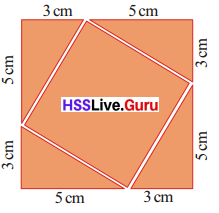What if we cut off triangles as in the picture below?What is the area of the remaining square?
Can we cut out a square of area 50 square centimetres from the large square?
How about a square of area 44$$\frac{1}{2}$$ square centimetres?

Yes, we can cut out a square of area 50 square centimetres from the large square as big square area is 64 square cm.
Yes, area 44$$\frac{1}{2}$$ square cm can also be cut from big square.
Explanation:
Side of the big square = 8 cm.
Area of big square = Side of the big square × Side of the big square
= 8 × 8
= 64 square cm.
Area of right triangle = (Side × Height) $$\frac{1}{2}$$
= 2 × 6 × $$\frac{1}{2}$$
= 1 × 6 × $$\frac{1}{1}$$
= 6 square cm.
Area of the small square = Area of big square – Area of right triangle
= 64 – 6
= 58 square cm.Right triangles

We have seen two ways of drawing a square of area 34 square centimetres:In both pictures, a side of such a square is the longest side of a right triangle
What is the relation between the lengths of the perpendicular sides of the triangle and area of the square?

There is a direct relationship between the lengths of the perpendicular sides of the triangle and area of the square as it helps to find the area of the small square.

Explanation:
Lengths of the perpendicular sides of the right angled triangle are the base and height of the triangle, which help to find the area of the square formed by the longest side of a right triangle.

Using GeoGebra, we can verify the relation between the areas of squares drawn on the three sides of a right triangle.
Draw a line AB and the perpendicular through A as below.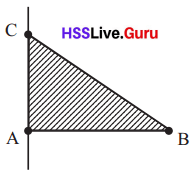Mark a point C on the perpendicular.
Now we can slide the line AC.
Using the Polygon tool, draw triangle ABC. Then using the Regular Polygon tool, we can draw squares on the sides AB, BC and AC, By selecting Area tool and clicking within the squares, we can see their areas.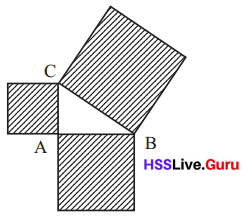What is the relation between the areas? Drag the vertices of the triangle and check. Does the relation between areas change? To make the area of the largest square 25, what should be the sides of the smaller squares?
Suppose we want the largest square to have area 41?
We cannot draw the largest square to have area 41 with the sides of 3cm and 4cm.

Explanation:
Area of the largest square = 25 square cm.
Side of largest square = 5 cm.
Side of smaller squares can be of 3cm and 4cm because 32 + 42 = 52

Pythagoras Textbook Page No. 171

We don’t know much about Pythagoras, a famous mathematician of ancient times. He was born around 570 BC in the island of Samos in Greece.
History says, in his youth he studied in Egypt and later came back to Greece to start a school. He taught that:
“The real nature of things could be understood only through Mathematics”
The picture shows a statue of Pythagoras at Samos, his place of birth.Indian Math

Certain geometric texts of ancient India are collectively termed sulvastura.
They are written by various authors at different periods estimated to be written around 500 BC.

In the Baudhayana sulvasutra, method of doubling a square is given:
By stretching a rope along the diagonal of a square, another square of double the area can be made.

In the Katyana sulvasutra, written around 200 BC, a more general construction is described:

By stretching a rope along the
diagonal of a rectangle, the sum
of the areas of square make by
the horiztontal and vertical sides.

The word sulva in Sanskrit means string or rope. The word sutra also means the same, but it also used for shortened statements of general principles.

Pythagoras relation Textbook Page No. 173

The square of the longest side of a right triangle is equal to the sum of the squares of the other two sides
Putting it in reverse, if the square of the longest side of a triangle is equal to the sum of the squares of the other two sides, then it is a right triangle.

That is, the property of having the square of one side equal to the sum of the squares of the other two, is peculiar to right triangles.
For example, since 32 + 42 = 52, a triangle with lengths of sides 3, 4, 5 is right. What if the lengths of sides are 6, 8, 10?

Different uses

We can use Pythagoras Theorem to make one large square from two smaller ones and to draw squares of specified areas.
We can also use it to construct perpendiculars and to verify perpendicularity.
For example, look at these lines:To check whether they are perpendicular to each other, mark a point on the horizontal line, 3 centimetres from the point where the lines meet; and a point on the vertical line, 4 centimetres up.If the distance between these points is 5 centimetres, then the lines are perpendicular; otherwise not.Pythagorean triples Textbook Page No. 175

The sum of the squares of two natural numbers may not be the square of another natural number.
12 + 22 = 5
But we do have
32 + 42 = 25 = 52
52+ 122 = 169 = 132
82 + 152 = 289 = 172
and so on.
Any three natural numbers, with the sum of the squares of two of them equal to the square of the third, is called a Pythagorean triple.
Some examples of Pythagorean
3, 4, 5
5, 12, 13
8, 15, 17
Can you find some more?

Some other examples of Pythagorean are (20, 21, 29) and (9, 40, 41).

Explanation:
Some examples of Pythagorean:
202+ 212 = 400 + 441 = 841 = 292
92+ 402 = 81 + 1600 = 1681 = 412

Doubling a square Textbook Page No. 158

Cut out two squares of the same size from thick paper: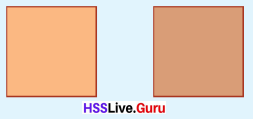These are to be cut and the pieces arranged to form one large square.
It can be done like this.
First cut along the diagonals’ of one square to make four triangles: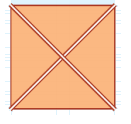Flip all these triangles outwards: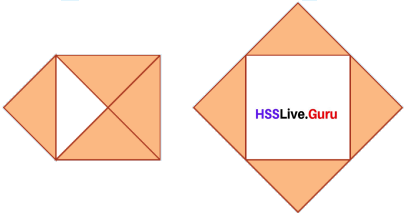Now place the uncut square in the vacant spot in the middle: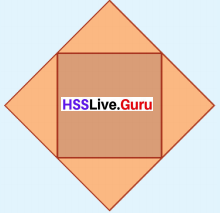Why does the second square fit inside exactly?
Suppose the sides of the first two squares are all 2 centimetres long. What is the area of each of these?
So, what is the area of the final large square?
Now let’s do this with two squares of area 9 square centimetres each. To make a square of area 9 square centimetres, how much should be the length of the sides?
Cut out two such squares and cut one of these as above to make a single large square. What is its area?
How do you make a square of area 50 square centimetres?
What about a square of area 32 square centimetres?

To make a square of 50 square cm, side of the square as to be 7.07 cm and 32 square cm the side of square to be 5.65 cm.

Explanation:
Area of each two squares = 9 square cm.
=> Side × Side = Area of square.
Side of the square = 3 cm.
Area of square = 50 square centimeters.
=> Side × Side = Area of square.
=> √50 = 7.07 cm.
Area of square = 32 square centimeters.
=> Side × Side = Area of square.
=> √32 = 5.65 cm.Making larger

We have seen that by cutting a square along the diagonals and placing the pieces around another square of the same size, we can make a square of double the size.
Now let’s cut in a different way.
Cut out a square of side 5 centimetres.
Instead of joining opposite corners to draw diagonals, mark points 1 centimetre away from corners and join:Cut along these lines and flip the four pieces outwards:Slide the left piece a little downwards, the right piece towards the left, and the top piece left and down to make a
large outer square with a small square hole inside as below: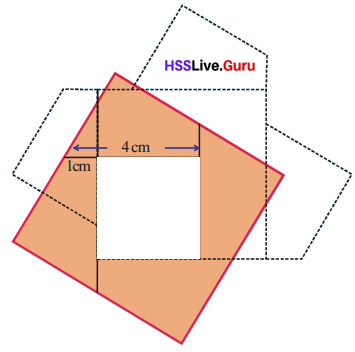What is the length of the sides of the inner square?
So, if we cut out a square of side 3 centimetres, we can fit it exactly into the hole.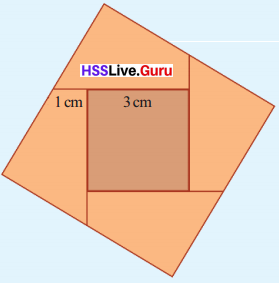It was the first square that was cut into four pieces. So the sum of the areas of these four pieces is equal to the area of the first square; that is 52 = 25 square centimetres.
What about the area of the second square placed inside?
So, the area of the final large square is
52 + 32 = 34 sq.cm.
Now cut out another square of side 5 centimetres again and join points marked 2 centimetres off the corners:If we cut along these lines and flip the four pieces outwards it would be like this:Let’s slide the pieces as before to make a square with a hole:What should be the length of the sides of the square needed to plug this hole?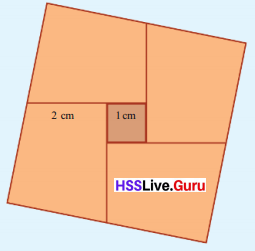What is the area of the filled up large square?
52 + 12 = 26 sq.cm

Again cut out a square of 5 centimetres and join points 1.5 centimetres off the corners. Cut out along these lines and rearrange the pieces as above.
What should be the length of the sides of the square to be placed inside?
What is the area of the filled up large square?

Area of the filled square = 36 square cm.

Explanation:
Side of the big square = 5 + 1.5 = 6.5 cm
Area of big square = Side of the big square × Side of the big square
= 6.5 × 6.5
= 42.25 square cm.
Side of the filled square = 1 + 1.5 = 2.5 cm.
Area of the filled square = (6.5 × 6.5) – (2.5 × 2.5)
= 42.25 – 6.25
= 36 square cm.

Two squares Textbook Page No. 163

We cut a square of side 5 centimetres in various ways and joined with another square to make larger squares of different sizes: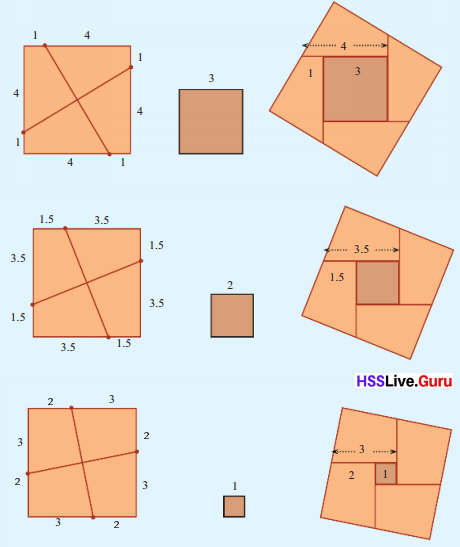To cut the first square into pieces, we mark points at the same distance from the corners. What is the relation between this distance and the length of the sides of the second square cut out to plug the hole?
Let’s look again at the way we made the large square.

When we mark points, we subtract the distance from the side of the first square. When we slide the pieces into position, we again subtract this distance. Thus, the side of the hole is the side of the original square reduced twice by the distance between the corners and the marks.

So, suppose we cut a square of side 8 centimetres by marking points 3 centimetres from the corners?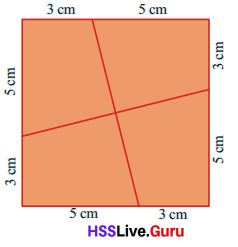If we cut along these lines and rearrange the pieces as before, the side of the square to fill the hole is.
8 – (2 × 3) = 2 cm
What is the area of this large square?

The area of this large square = 64 square cm.

Explanation:
Side of the big square = 8 cm.
Area of big square = Side of the big square × Side of the big square
= 8 × 8
= 64 square cm.Another question

We want to cut a square of side 8 centimetres as before and fit a square of side 6 centimetres within to make a
larger square. How should we cut the first square?
Twice the distance of the points to be marked on the first square from its corners subtracted from 8 centimeters should be 6 centimetres.
So, twice this distance is
8 – 6 = 2 cm
So this distance is half of 2 centimetres; that is 1 centimetre.

Cut out a square of side 8 centimetres, cut into pieces like this and rearrange as before. Isn’t the sides of the inner square to 6 centimetres?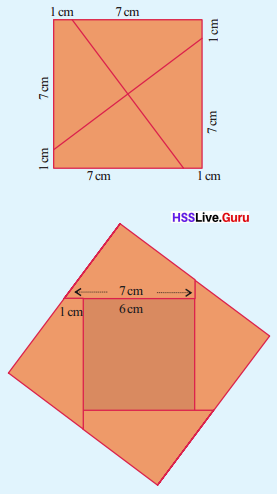What is the area of the final large square?
82 + 62 = 100 sq. cm

• Cut out a square of side 7 centimetres and another of side 3 centimetres. Cut the larger square suitably and join with the smaller square to make one large square.
What is the area of this square?
Area of the large square = 58 square cm.

Explanation:
Length of side of the square = 7 cm.
Length of side of the square = 3 cm.
Area of the large square = 72 + 32
=> 49 + 9
=> 58 square cm.

• Cut out a square of side 8 centimetres. Cut this suitably and join with a suitable square to make a large square of area 80 square centimetres.
Area of the large square = 80 square cm.

Explanation:
Length of side of the square = 8cm.
Area of the square = 80 square cm.
Length of side of the square = 4cm.
Area of the large square = 82 + 42
=> 64 + 16
=> 80 square cm.

• A square of side 9 centimetres is to be cut and joined with another square to make a large square of area 117 square centimetres. What should be the side of the second square? At what distance from the corners of the first square should points be marked for cutting it?

Side of other square = 6 cm.
3cm distance from the corners of the first square should points be marked for cutting it.

Explanation:
Area of the square = 117 square centimeters.
Side of one square = 9 cm.
Side of other square = ?? cm.
92 + 72 = 576 + 49 = 625 = 25 cm.
=> 81 + ??2 = 117
=> ??2 = 117 – 81
=> ??2 = 36.
=> ?? =  6 cm.

Drawing Squares Textbook Page No. 166

Remember how we joined together squares of sides 5 centimetres and 8 centimetres and made a large square?
First we find half the difference of the sides.
(5 – 3 ) ÷ 2 = 1
Then we mark points on the large square, 1 centimetre away from the corners. Cutting along the lines joining those points and rearranging the pieces with the small square inside, we get a square of area 25 + 9 = 34 square centimetres.If we just want to draw a square of this area, instead of actually making it, we need only draw a line equal in length to the side of the square. Let’s see how we do this.

Each of the four pieces got by cutting the square of side 5 centimetres have two sides of length 4 centimetres and I centimetre. By stacking the pieces, we can see that the other sides also are of equal lengths.

Now look at these pictures: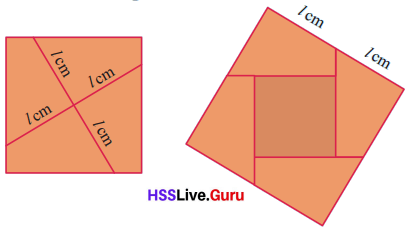The length of the sides of the final large square is equal to the length of the lines drawn to cut the first square.
So, we have an easy method to draw a side of a square of area 34 square centimetres.
First draw a square of side 5 centimetres, mark two points 1 centimetre away from two opposite corners and join
them: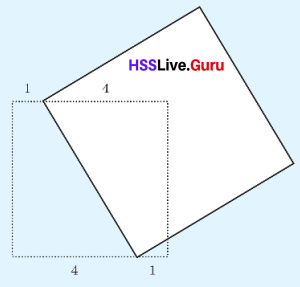The square drawn with this line as a side has area 34 square centimetres.

Instead of shifting 1 centimetre from each of the two corners we can shift 2 × 1 = 2 centimetres from one corner.Suppose we want to join a square of side 5 centimetres and another of side 1 centimetre and draw square of area 25 + 1 = 26 square centimetres. For this, we mark two points (5 – 1) ÷ 2 = 2 centimeters away from two opposite corners of the larger square and join them:Then we draw a square with this line as a side.
We can draw without taking half of 5 – 1 = 4. Instead of marking 2 centimetres on two sides, we mark 4 centimetres on one side.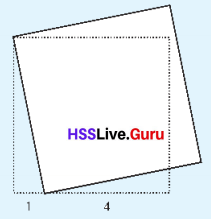Let’s have another look at the two squares we have drawn.In each figure, the side of the square is the longest side of a right triangle.
The sum of the areas of squares drawn on the other two sides of the triangle.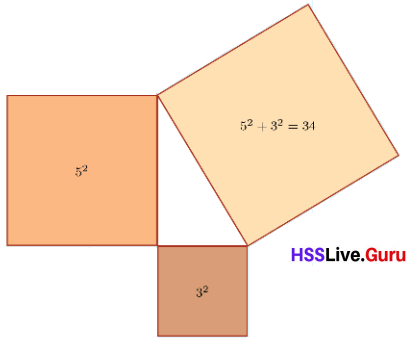Now draw a right triangle and squares on all three sides on thick paper: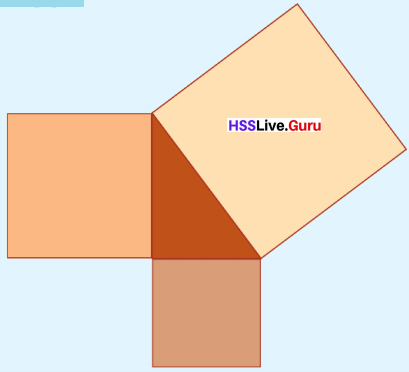In the medium size square, mark the point of intersection of diagonals; and through this point, mark lines parallel to the perpendicular sides of the largest square: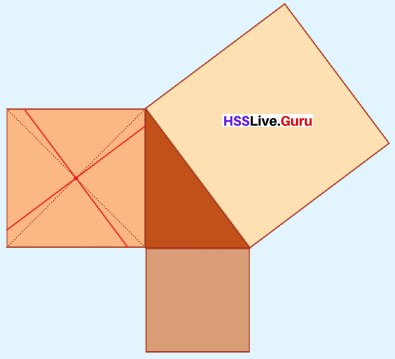Now cut along these lines to split the square iito four pieces. Cut out the smallest square also. Arrange all these inside the largest square as below:What do we see from all this?

The area of squares drawn out are equal to the area of the largest side of the triangle formed from cutting.

Explanation:
It is noticed that the area of squares drawn out are equal to the area of the largest side of the triangle formed from cutting.

The area of the square drawn on the largest side of a right triangle is equal to the sum of the areas of the squares drawn on the other two sides.
This is known as Pythagoras Theorem, in honour of the philosopher Pythagoras, who lived in Greece.
Using this, we can draw a square of area 10 square centimetres. We have
10 = 32 + 12
So according to Pythagoras Theorem, we need only draw a right triangle of perpendicular sides 3 centimetres and 1 centimetre and then draw a square on the longest side.How about a square of area 7 square centimeters?

It cannot be drawn the side of the square of having area of 7 square centimeters.

Explanation:
Area of 7 square centimeters cannot be drawn. So according to Pythagoras Theorem, we need only draw a right triangle of perpendicular sides 3 centimeters and 1 centimeters and then draw a square on the longest side.

7 cannot be written as the Sum of two perfect squares
But we have
7 = 42 – 32
So by Pythagoras Theorem, we need only draw a right triangle of longest side 4 centimetres and another side 3 centimetres.
How do we draw it?
First draw a 3 centimetre long line and the perpendicular at one end:Now use a compass to mark a point on the perpendicular, at a distance of 4 centimetres from the other end of the first line.The area of the square in the vertical side of this triangle is 42 – 32 = 7 square centimetres, according to Pythagoras Theorem.Can’t you now draw squares of areas given below?

• 20 square centimeters.
Yes, it can be done for  area of the square = 20 square centimeters.

Explanation:
Area of the square = 20 square centimeters.
42 + 22 = 16 + 4 = 20.
Sides of the squares = 4cm , 2cm.

• 39 square centimeters
No, it cannot be drawn a square of having 39 square centimeters.

Explanation:
Area of the square = 39 square centimeters.

• 40 square centimeters.
Yes, it can be done a square of having 40 square centimeters.

Explanation:
Area of the square = 40 square centimeters.
62 + 22 = 36 + 4 = 40.
Sides of the squares = 6cm , 2cm.

• 65 square centimeters
Yes, it can be drawn a square of having 65 square centimeters.

Explanation:
Area of the square = 65 square centimeters.
72 + 42 = 49 + 16 = 65.
Sides of the squares = 7cm , 4cm.Squared relation Textbook Page No. 173

We can state Pythagoras Theorem as a relation between the lengths of the sides of a right triangle. The longest side of a right triangle is called its hypotenuse.

The square of the hypotenuse of a right triangle is equal to the sum of the squares of the other two sides.
For example, if the length of the perpendicular sides of a right triangle are 3 centimetres and 4 centimetres, then the square of the hypotenuse is
32 + 42 = 25
and so the length of the hypotenuse is 5 centimetres.Look at this problem: What is the length of the diagonal of the rectangle below?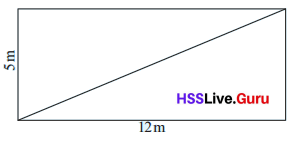The diagonal of the rectangle is the hypotenuse of a right triangle :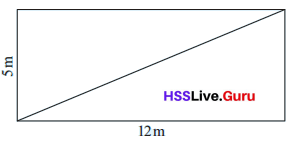So, the square of the diagonal is
52 + 122 = 169
and the length of the diagonal is
$$\sqrt{169}$$ = 13 m

• What is the length of the fourth side of the quadrilateral shown below?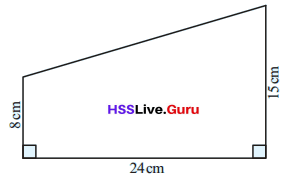Length of the fourth side of the quadrilateral shown below = 25 cm.

Explanation:
Draw a line to side 24cm.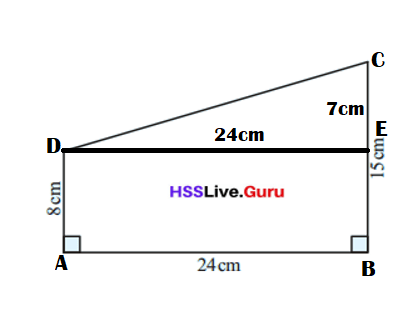Length of the other side = 242 + 72 = 576 + 49 = 625 = 25 cm.

• What is the area of the triangle shown below?Area of the big triangle = 175 square cm.

Explanation:
Area of the small triangle = (Base × height) ÷ 2
Height of triangle = 252 – 72 = 625 – 49 = 576 = 24 cm.
Base of triangle = 7 cm.
Area of the 1 triangle = (Base × height) ÷ 2
= (7 × 24) ÷ 2
= 168 ÷ 2
= 84 square cm.

Area of the 2 triangle = (Base × height) ÷ 2
= (7 × 26) ÷ 2
= 182 ÷ 2
= 91 square cm.

Area of the  big triangle = Area of the 1 triangle + Area of the 2 triangle
= 84 + 91
= 175 square cm.

• The picture shows a plot of land in the form of a right angled triangle joined to a square.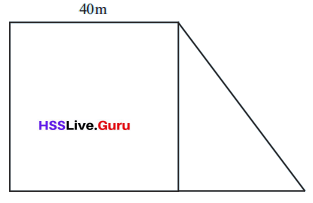The total area of the plot is 2200 square meters. What is its perimeter’?
Perimeter of figure = 280 m.

Explanation:
Total area of the plot = 2200 square meters.
Side of the square = 40 m.
Perimeter of the square = 4 × side of the square
= 4 × 40
= 160 meters.
Base of triangle = 40 m.
Height of triangle = 40 m.
Perimeter of the triangle = side + side + side
= 40 + 40 + 40
= 80 + 40
= 120 m.
Perimeter of figure = Perimeter of the square  + Perimeter of the triangle
= 160 + 120
= 280 m.

error: Content is protected !!